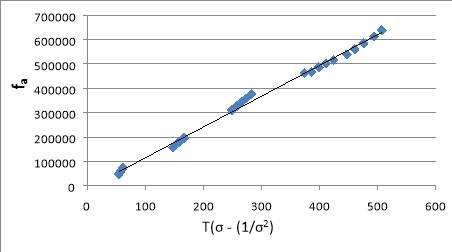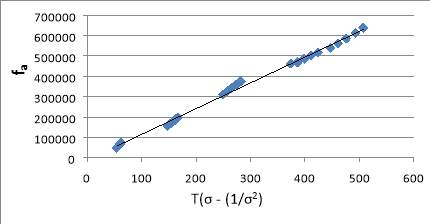Disclaimer: This is an example of a student written essay.

Any scientific information contained within this essay should not be treated as fact, this content is to be used for educational purposes only and may contain factual inaccuracies or be out of date.

# Determining Fraction of Cross-Linked Units in Isoprene

 ✅ Paper Type: Free Essay ✅ Subject: Chemistry ✅ Wordcount: 3369 words ✅ Published: 18th May 2020

Abstract.  The objective of the present work is to demonstrate an experimental methodology to determine the fraction of cross-linked units in isoprene. For this purpose isoprene was stretched out at a constant elongation. For each elongation, the experimental effort ranged of temperature for the region 303-344 K to evaluate the force. Then calculated effective Young’s modulus, so can be obtained the average of cross-link fraction between polymer molecules. It is shown that once the temperature was dropped, the weight was decreased. The effect of higher temperature had on the elasticity of the isoprene was amplified under more weight. Therefore, the change of temperature may be due to effect to stress, which has affected the elastic property of elastomer. Then crosslink fraction can be evaluated which is 0.01.

If you need assistance with writing your essay, our professional essay writing service is here to help!

## Introduction

Elastomeric materials are applied widely to demanding applications on account of their inherent reversible deformation behavior. The chains of molecules in elastomer have a natural elasticity: Elastomer will be stretched after acquiring the force, once remove the force elastomer spring back to the original length. The molecular chains of an elastomer principally perform as springs which are called crosslinks. Furthermore, these crosslinks and the elastomers will break if obtain too much force.

When informing the tensile strength of materials loaded, it is common to consider forces in term of the stress (the force by the cross-sectional area):

fa = $\frac{\mathrm{F}}{\mathrm{A}}$

(1)

where fa is the stress, the stress is attained by using the measured force (F) and the original material area. A statistical mechanical treatment of elasticity, based on a model which assumes that the polymer chains are freely jointed and that the distance between the ends of a given polymer chain is characterised by a random distribution, can be written algebraically as:

${\mathrm{f}}_{\mathrm{a}}=\left(\frac{\mathrm{\rho RT}}{\mathrm{zM}}\right)\left(\mathrm{\sigma }–\frac{1}{{\mathrm{\sigma }}^{2}}\right)=\mathrm{Y}\left[\mathrm{T}\left(\mathrm{\sigma }–\frac{1}{{\mathrm{\sigma }}^{2}}\right)\right]$

(2)

Here M is a molar mass of monomer that has relative with a density of the polymer (ρ), z is the average number of monomer units between crosslinks and σ is the fractional elongation which evaluated from σ = L/L0. Calculate an effective Young’s modulus by plotting fa against T(σ – (1/σ2). Thus z can be attained and then use this equation Fcl = $\frac{\mathrm{n}}{\mathrm{N}}$

= $\frac{1}{\mathrm{z}}$

to attain the fraction of cross-links.

## Experimental

1. Turned on the balance and unscrewed the set-screws with the shaft at the bottom of the sample for balance. Measured the sample length. Turned on the tap for compressed air. Set the air flow between 9 and 10 with the bench tap.

2. Set the temperature controller to a temperature between 70 and 80 °C. Wait a few minutes until the temperature was stable. Tared the balance. Expanded the sample approximately 10-20% by pulled the connecting shaft down and then screwed the set-screw to the shaft. Took a note from the digital thermometer when the measurement was stable.

3. Reduced the temperature between 5 to 10 °C. Noted the results when the measurement was stable. Continued this process until the temperature was between 20 and 30 °C. Unscrewed the set-screws and then expanded the sample again approximately 10-20%. Performed sets of measurements in which the temperature was risen to between 70 and 80 °C.

4. Repeated the above to get about 5 sets. Recorded the width and thickness of the sample. After the experiment was finished, turned off the heater controller, air flow and balance.

## Results and discussion

The elastomer sample is isoprene (2-methylbutadiene) which has thickness 0.09 mm, width 6.6 mm, original length 23.5 mm and molar mass 68.12 g/mol. In this experiment assume the density of the elastomer is 0.98 g/cm3.

Table 1.  Length stretched 25 mm by connecting rod, σ = 1.06

 Temperature (K) Weight (kg) fa (Pa) 343.05 -0.0045 74318.18 332.85 -0.00407 67216.67 323.15 -0.00371 61271.21 313.05 -0.00332 54830.30 303.05 -0.00294 48554.55

Table 2. Length stretched 28 mm by connecting rod, σ = 1.19

 Temperature (K) Weight (kg) fa (Pa) 303.05 -0.00946 156233.33 313.05 -0.01014 167463.64 322.95 -0.0108 178363.64 333.25 -0.01135 187446.97 343.35 -0.01194 197190.91

Table 3. Length stretched 32 mm by connecting rod, σ = 1.36

 Temperature (K) Weight (kg) fa (Pa) 343.05 -0.02278 376215.15 333.05 -0.0217 358378.79 322.85 -0.02082 343845.45 313.15 -0.01978 326669.70 303.15 -0.01884 311145.45

Table 4. Length stretched 38 mm by connecting rod, σ = 1.62

 Temperature (K) Weight (kg) fa (Pa) 303.05 -0.02801 462589.39 312.75 -0.02834 468039.39 323.05 -0.02947 486701.52 333.25 -0.03041 502225.76 343.65 -0.03132 517254.55

Table 5. Length stretched 42 mm by connecting rod, σ = 1.79

 Temperature (K) Weight (kg) fa (Pa) 344.15 -0.0387 639136.36 334.55 -0.03711 612877.27 322.85 -0.03545 585462.12 312.35 -0.03397 561019.70 303.25 -0.03269 539880.30

Plot fa against T $\left(\mathrm{\sigma }–\frac{1}{{\mathrm{\sigma }}^{2}}\right)$

via equation 2, ${\mathrm{f}}_{\mathrm{a}}=\left(\frac{\mathrm{\rho RT}}{\mathrm{zM}}\right)\left(\mathrm{\sigma }–\frac{1}{{\mathrm{\sigma }}^{2}}\right)=\mathrm{Y}\left[\mathrm{T}\left(\mathrm{\sigma }–\frac{1}{{\mathrm{\sigma }}^{2}}\right)\right]$Figure 1. Sample graph between fa and T (σ – (1/σ2)

Can be obtained Y from a slope in figure 1, so  Y = 1264.9

Then calculated z from equation 2, so   z = 94.56

And calculated the fraction of cross-links (Fcl):

Fcl = $\frac{1}{z}$

= 0.011

As noted in the results sections, once the temperature was dropped, the weight was decreased as shown in table 1, 3 and 5. In addition, higher temperatures also affect more weight of the elastomer because the molecules are more excited and want to be in a random state as given in table 2 and 4. Therefore, the change of temperature may be due to effect to stress, which has affected the elastic property of elastomer. Moreover, during recorded the values shown that there was a fluctuation of the weight because the force is trying to pull the elastomer back to its equilibrium length. By looking at figure 1, according to calculate the equation, the crosslink fraction is 0.01 that means approximately 100 molecules of isoprene have one crosslink in the polymer.

Our academic experts are ready and waiting to assist with any writing project you may have. From simple essay plans, through to full dissertations, you can guarantee we have a service perfectly matched to your needs.

## Conclusions

The applied experimental methodology for determining the fraction of cross-linked units in isoprene (2-methylbutadiene). Isoprene was stretched out at a constant elongation. The results also showed that once the temperature was dropped, the weight was decreased. The effect of higher temperature had on the elasticity of the isoprene was amplified under more weight. When isoprene was heated, the molecules stretched out, making them more excited and able to be in a random state. Therefore, the change of temperature may be due to effect to stress, which has affected the elastic property of elastomer. Constitutive equations are evaluated the crosslink fraction which is 0.01 that means approximately 100 molecules of isoprene will have one crosslink in the polymer. The balance led to some of the sources of error. After tested 3 sets of temperature, the weight values have fluctuated. Hence, the teacher assistant moved the balance to reduce this error. However, there was still fluctuation weight error because the force is trying to pull the elastomer back to its equilibrium length. If have the chance to conduct this experiment again, an experiment should test more range of temperature such as 273 – 373 K to observe other property changes.

## References

1   Polymerization, S. et al. (2016) ‘Communications Independent Control of Elastomer Properties through Stereocontrolled Synthesis’, pp. 13076–13080.

2   Roylance, D. (2008) ‘Mechanical properties of materials’. pp. 1-128.

3   Technologies, A. and Composites, P. (2020) ‘POLYMER’. pp. 1-43.

4   Urry, D. W., Harris, D. and Prasad, U. (1984) ‘B 1984 Academic Press, Inc.’, 125(3), pp. 1082–1088.

5   Engineering, P. and Collection, S. P. (1994) ‘The effect of annealing on stretched trans-1 , 4-polyisoprene networks’. pp. 290-300.

## Appendices

The elastomer sample is isoprene (2-methylbutadiene) which has thickness 0.09 mm, width 6.6 mm, original length 23.5 mm and molar mass 68.12 g/mol. In this experiment assume the density of the elastomer is 0.98 g/cm3.

The calculation example; Temperature 343.05 K, weight -4.5g and stretched length 25 mm Calculate Force (F) from                                           F = mg

So      F = (-0.0045 kg) x (-9.81 m/s2)

F = 0.044145 N

F ≈ 0.044 N

Then, calculate Force per unit area (fa): fa = $\frac{\mathrm{F}}{\mathrm{A}}$

fa =

fa = 74318.18182 Pa

fa ≈ 74318.18 Pa

And      σ = $\frac{\mathrm{L}}{{\mathrm{L}}_{0}}$

σ =

σ = 1.063829787

σ ≈ 1.06Plot fa against T $\left(\mathrm{\sigma }–\frac{1}{{\mathrm{\sigma }}^{2}}\right)$

via equation 2  ${\mathrm{f}}_{\mathrm{a}}=\left(\frac{\mathrm{\rho RT}}{\mathrm{zM}}\right)\left(\mathrm{\sigma }–\frac{1}{{\mathrm{\sigma }}^{2}}\right)=\mathrm{Y}\left[\mathrm{T}\left(\mathrm{\sigma }–\frac{1}{{\mathrm{\sigma }}^{2}}\right)\right]$

Figure 2. Sample graph between fa and T(σ – (1/σ2)

Can obtain Y from slope in figure 2, so   Y = 1264.9

Then calculate z from equation 2, so   z = 94.55952109

z ≈ 94.56

And calculate the fraction of cross-links (Fcl):

Fcl = $\frac{1}{z}$

= 0.01057535 ≈ 0.011

NB. Density of the elastomer ( $\mathrm{\rho }$

)  = 980 kg/m3

Molar mass (M)  = 0.06812 kg/mol

Ideal gas constant (R)  = 8.314 m3•atm/mol•K

Polymerization, S. et al. (2016) ‘Communications Independent Control of Elastomer Properties through Stereocontrolled Synthesis’, pp. 13076–13080.

 Roylance, D. (2008) ‘Mechanical properties of materials’.

 Technologies, A. and Composites, P. (2020) ‘POLYMER’.

 Urry, D. W., Harris, D. and Prasad, U. (1984) ‘B 1984 Academic Press, Inc.’, 125(3), pp. 1082–1088.

 Engineering, P. and Collection, S. P. (1994) ‘The effect of annealing on stretched trans-1 , 4-polyisoprene networks’.

View all

## DMCA / Removal Request

If you are the original writer of this essay and no longer wish to have your work published on UKEssays.com then please:

Related Services

Related Lectures